An algebraic expression is a legal combination of operators and operands. Operand is the quantity on which a mathematical operation is performed. Operand may be a variable like x, y, z or a constant like 5, 4, 6 etc. Operator is a symbol which signifies a mathematical or logical operation between the operands. Examples of familiar operators include +, -, *, /, ^ etc.

An algebraic expression can be represented using three different notations. They are infix, postfix and prefix notations:

Infix: It is the form of an arithmetic expression in which we fix (place) the arithmetic operator in between the two operands.

Example: (A + B) * (C – D)

Prefix: It is the form of an arithmetic notation in which we fix (place) the arithmetic operator before (pre) its two operands. The prefix notation is called as polish notation (due to the polish mathematician Jan Lukasiewicz in the year 1920).

Example: * + A B – C D

Postfix: It is the form of an arithmetic expression in which we fix (place) the arithmetic operator after (post) its two operands. The postfix notation is called as suffix notation and is also referred to reverse polish notation.

Example: A B + C D – *

The three important features of postfix expression are:

1.  The operands maintain the same order as in the equivalent infix expression.
2. The parentheses are not needed to designate the expression unambiguously.
3. While evaluating the postfix expression the priority of the operators is no longer relevant

We consider five binary operations: +, -, *, / and \$ or ↑ (exponentiation). For these binary operations, the following in the order of precedence (highest to lowest):Converting expressions using Stack:

Let us convert the expressions from one type to another. These can be done as follows:

1. Infix to postfix
2. Infix to prefix
3. Postfix to infix
4. Postfix to prefix
5. Prefix to infix
6. Prefix to postfix

Conversion from infix to postfix:

Procedure to convert from infix expression to postfix expression is as follows:

1. 1. Scan the infix expression from left to right.
2. If the scanned symbol is left parenthesis, push it onto the stack.
3. If the scanned symbol is an operand, then place directly in the postfix expression (output).
4. If the symbol scanned is a right parenthesis, then go on popping all the items from the stack and place them in the postfix expression till we get the matching left parenthesis.
5. If the scanned symbol is an operator, then go on removing all the operators from the stack and place them in the postfix expression, if and only if the precedence of the operator which is on the top of the stack is greater than (or greater than or equal) to the precedence of the scanned operator and push the scanned operator onto the stack otherwise, push the scanned operator onto the stack.

Example 1:
Convert ((A – (B + C)) * D) ↑ (E + F) infix expression to postfix form:Conversion from infix to prefix:

The precedence rules for converting an expression from infix to prefix are identical. The only change from postfix conversion is that traverse the expression from right to left and the operator is placed before the operands rather than after them. The prefix form of a complex expression is not the mirror image of the postfix form.

Example 1:
Convert the infix expression A + B – C into prefix expression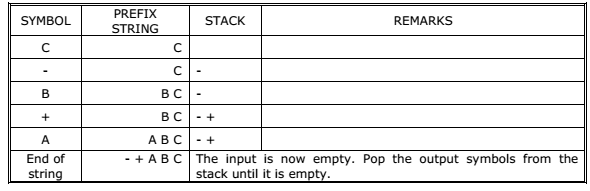Conversion from postfix to infix:

Procedure to convert postfix expression to infix expression is as follows:

1.  Scan the postfix expression from left to right.
2.  If the scanned symbol is an operand, then push it onto the stack.
3.  If the scanned symbol is an operator, pop two symbols from the stack and create it as a string by placing the operator in between the operands and push it onto the stack.
4. Repeat steps 2 and 3 till the end of the expression.

Example:
Convert the following postfix expression A B C * D E F ^ / G * – H * + into its
equivalent infix expression.Conversion from postfix to prefix:

Procedure to convert postfix expression to prefix expression is as follows:

1.  Scan the postfix expression from left to right.
2. If the scanned symbol is an operand, then push it onto the stack.
3. If the scanned symbol is an operator, pop two symbols from the stack and create it as a string by placing the operator in front of the operands and push it onto the stack.
4. Repeat steps 2 and 3 till the end of the expression.

Example:
Convert the following postfix expression A B C * D E F ^ / G * – H * + into its
equivalent prefix expression.Conversion from prefix to infix:

Procedure to convert prefix expression to infix expression is as follows:

1.  Scan the prefix expression from right to left (reverse order).
2. If the scanned symbol is an operand, then push it onto the stack.
3. If the scanned symbol is an operator, pop two symbols from the stack and create it as a string by placing the operator in between the operands and push it onto the stack.
4. Repeat steps 2 and 3 till the end of the expression.

Example:
Convert the following prefix expression + A * – * B C * / D ^ E F G H into its equivalent
infix expression.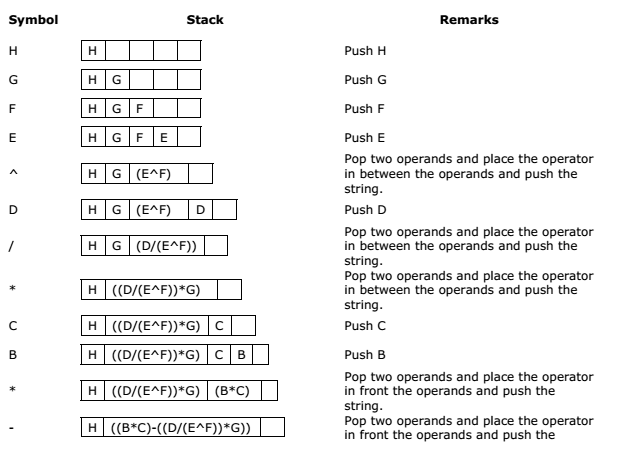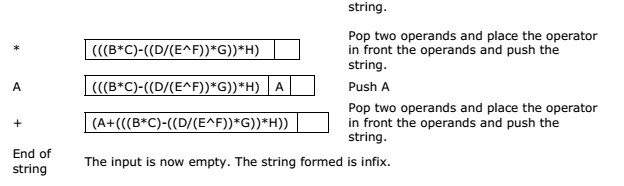Conversion from prefix to postfix:

Procedure to convert prefix expression to postfix expression is as follows:

1.  Scan the prefix expression from right to left (reverse order).
2. If the scanned symbol is an operand, then push it onto the stack.
3. If the scanned symbol is an operator, pop two symbols from the stack and create it as a string by placing the operator after the operands and push it onto the stack.
4. Repeat steps 2 and 3 till the end of the expression.

Example:
Convert the following prefix expression + A * – * B C * / D ^ E F G H into its equivalent
postfix expression.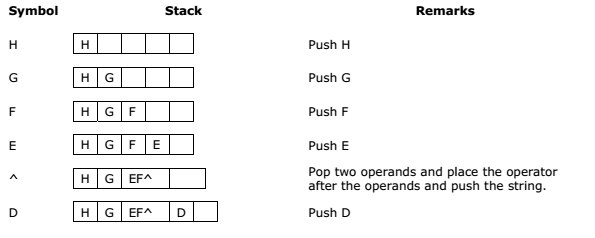Evaluation of postfix expression:

The postfix expression is evaluated easily by the use of a stack. When a number is seen, it is pushed onto the stack; when an operator is seen, the operator is applied to the two numbers that are popped from the stack and the result is pushed onto the stack. When an expression is given in postfix notation, there is no need to know any precedence rules; this is our obvious advantage

Example 1: Evaluate the following postfix expression: 6 2 3 + – 3 8 2 / + * 2 ↑ 3 +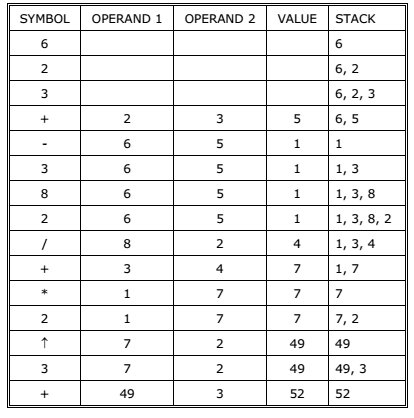Applications of stacks:

1.  Stack is used by compilers to check for balancing of parentheses, brackets and braces.
2. Stack is used to evaluate a postfix expression.
3. Stack is used to convert an infix expression into postfix/prefix form.
4. In recursion, all intermediate arguments and return values are stored on the processor’s stack.
5. During a function call the return address and arguments are pushed onto a stack and on return they are popped off.

You may be interested in:
Data Structures and Algorithms – MCQs.
Data Structures and Algorithms Online Tests